# pdeviz

Create and plot PDE visualization object

Since R2021a

## Syntax

``pdeviz(MeshData,NodalData)``
``pdeviz(MeshData)``
``pdeviz(___,Name,Value)``
``pdeviz(figure,___)``
``V = pdeviz(___)``

## Description

example

````pdeviz(MeshData,NodalData)` creates a `PDEVisualization` object and plots the data at the mesh nodes as a surface plot. For details, see PDEVisualization Properties.```

example

````pdeviz(MeshData)` creates a `PDEVisualization` object and plots the mesh.```

example

````pdeviz(___,Name,Value)` customizes the plot appearance using one or more `Name,Value` arguments. Use name-value arguments with any combination of arguments from the previous syntaxes.```
````pdeviz(figure,___)` specifies the graphics container for the `PDEVisualization` object. For example, you can plot the object in Figure 3 by specifying `pdeviz(figure(3), ___)`.```

example

````V = pdeviz(___)` returns a handle to the `PDEVisualization` object, using any of the previous syntaxes.```

## Examples

collapse all

Use the `pdeviz` function to create a PDE visualization object and plot it. Change the properties of this object to interact with the resulting plot.

Create a structural analysis model for a 3-D problem.

`structuralmodel = createpde("structural","static-solid");`

Import the beam geometry and plot it.

```importGeometry(structuralmodel,"SquareBeam.stl"); pdegplot(structuralmodel,"FaceLabels","on","FaceAlpha",0.5)```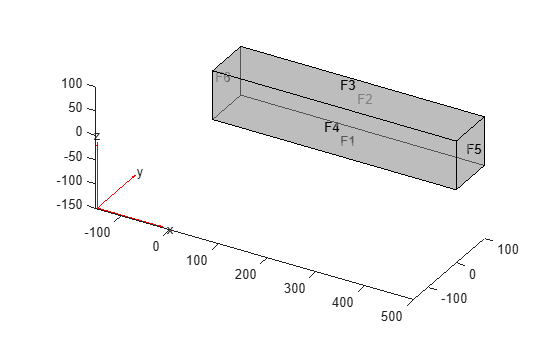Specify Young's modulus and Poisson's ratio.

```structuralProperties(structuralmodel,"PoissonsRatio",0.3, ... "YoungsModulus",210E3);```

Specify that face 6 is a fixed boundary.

`structuralBC(structuralmodel,"Face",6,"Constraint","fixed");`

Specify the surface traction for face 5.

```structuralBoundaryLoad(structuralmodel,"Face",5, ... "SurfaceTraction",[0;0;-2]);```

Generate a mesh and solve the problem.

```msh = generateMesh(structuralmodel); structuralresults = solve(structuralmodel);```

Call `pdeviz` with only the mesh data. This call creates a `PDEVisualization` object and plots the mesh.

```figure v = pdeviz(msh)```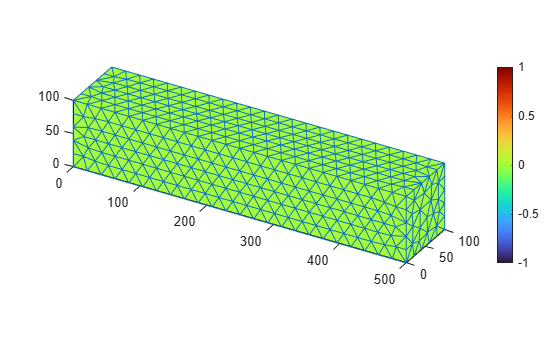```v = PDEVisualization with properties: MeshData: [1x1 FEMesh] NodalData: [0x1 double] MeshVisible: on Transparency: 1 Position: [0.1300 0.1100 0.6716 0.8150] Units: 'normalized' Show all properties ```

Update the plot by adding the von Mises stress as the `NodalData` property of the `PDEVisualization` object v. The plot now shows the von Mises stress and the mesh.

```figure v.NodalData = structuralresults.VonMisesStress;```

Update the plot by adding the displacement as the `DeformationData` property of the `PDEVisualization` object v. The plot shows the deformed shape with the von Mises stress.

```figure v.DeformationData = structuralresults.Displacement;```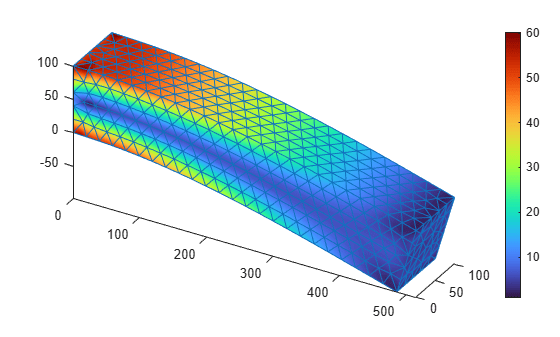Update the plot to hide the mesh.

```figure v.MeshVisible = "off";```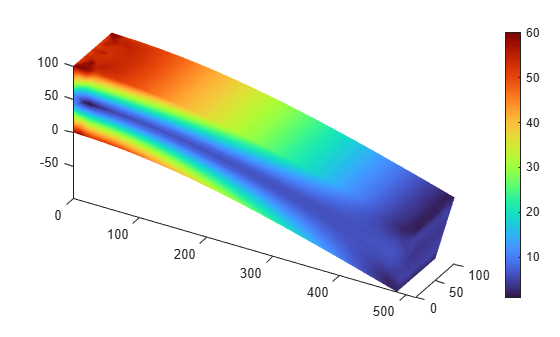Update the plot to hide the axes.

```figure v.AxesVisible = "off";```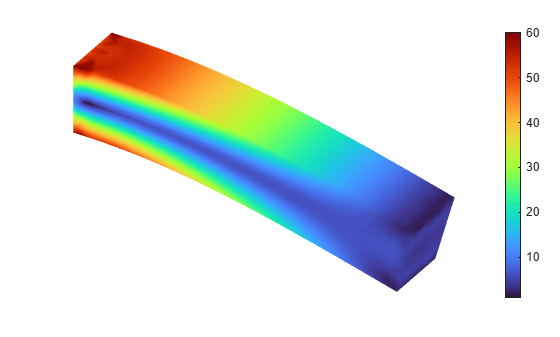## Input Arguments

collapse all

Finite element mesh, specified as an FEMesh Properties object.

Data at mesh nodes, specified as a column vector.

Data Types: `double`

### Name-Value Arguments

Specify optional pairs of arguments as `Name1=Value1,...,NameN=ValueN`, where `Name` is the argument name and `Value` is the corresponding value. Name-value arguments must appear after other arguments, but the order of the pairs does not matter.

Before R2021a, use commas to separate each name and value, and enclose `Name` in quotes.

Example: `pdeviz(model.Mesh,results.NodalSolution,"MeshVisible","on","DeformationData",results.Displacement)`

Surface transparency, specified as a real number from `0` through `1`. The default value `1` indicates no transparency. The value `0` indicates complete transparency.

Data Types: `double`

Toggle to show mesh, specified as `"on"` or `"off"`, or as numeric or logical `1` (`true`) or `0` (`false`). A value of `"on"` is equivalent to `true`, and `"off"` is equivalent to `false`. Thus, you can use the value of this argument as a logical value.

When plotting only the mesh, the default is `"on"`. Otherwise, the default is `"off"`.

Data Types: `char` | `string`

Mesh deformation data, specified as one of the following:

• An `FEStruct` object with the properties `ux`, `uy`, and, for a 3-D geometry, `uz`

• A structure array with the fields `ux`, `uy`, and, for a 3-D geometry, `uz`

• A matrix with either two columns for a 2-D geometry or three columns for a 3-D geometry

Level of mesh deformation, specified as a nonnegative number. Use this name-value argument together with `DeformationData`.

`pdeviz` computes the default value of `DeformationScaleFactor` based on the mesh and the value of `DeformationData`.

Data Types: `double`

Toggle to hide or show axes, specified as `"on"` or `"off"`, or as numeric or logical `1` (`true`) or `0` (`false`). A value of `"on"` is equivalent to `true`, and `"off"` is equivalent to `false`. Thus, you can use the value of this argument as a logical value.

Background color, specified as an RGB triplet, a hexadecimal color code, a color name, or a short name.

Colorbar visibility, specified as `"on"` or `"off"`, or as numeric or logical `1` (`true`) or `0` (`false`). A value of `"on"` is equivalent to `true`, and `"off"` is equivalent to `false`. Thus, you can use the value of this property as a logical value. The value is stored as an on/off logical value of type `matlab.lang.OnOffSwitchState`.

Color limits, specified as a two-element vector of the form ```[min max]```. The color limits indicate the color data values that map to the first and last colors in the colormap.

Chart title, specified as a character vector, cell array of character vectors, string array, or categorical array.

Example: `"My Title Text"`

To create a multi-line title, specify a cell array of character vectors or a string array. Each element in the array corresponds to a line of text.

Example: `{"My","Title"};`

If you specify the title as a categorical array, MATLAB® uses the values in the array, not the categories.

If you create the chart using tabular data, the default chart has an autogenerated title. If you do not want a title, specify `""`.

Azimuth and elevation of view, specified as a two-element vector of the form `[azimuth elevation]` defined in degree units. Alternatively, use the `view` function to set the view.

## Output Arguments

collapse all

Visualization container, returned as a handle to the `PDEVisualization` object. For details, see PDEVisualization Properties.

## Version History

Introduced in R2021a